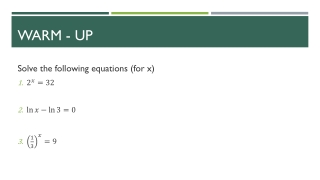DownloadDownload PresentationWarm - Up

# Warm - Up

Télécharger la présentation## Warm - Up

- - - - - - - - - - - - - - - - - - - - - - - - - - - E N D - - - - - - - - - - - - - - - - - - - - - - - - - - -
##### Presentation Transcript

1. Warm - Up Solve the following equations (for x)

2. Solving Exponential & Logarithmic Functions Section 3.4

3. Objectives Students will be able to… • Use log properties to solve exponential equations • Solve equations with variables in the exponents • Evaluate logs and natural logs • Use the change of base formula to evaluate logs with bases other than 10

4. Strategies for solving Exponential andLogarithmic Functions • Rewrite the original equation in a form that allows the use of the One-to-One Properties of exponential or logarithmic functions • Rewrite an exponential equation in logarithmic form and apply the Inverse Property of logarithmic functions ( and ) • Rewrite a logarithmic equation in exponential form and apply the Inverse Property of exponential functions. ( and )

5. Examples Solve each equation and approximate the result to three decimal places if necessary 1) 2)

6. Example 3) 4)

7. Practice Work on worksheet “3.2 Solving Exponential Equations”

8. Homework Finish 3.4 Solving Exponential Equations Worksheet

9. Closure On the note card provided, solve the following function(hand in on way out the door)